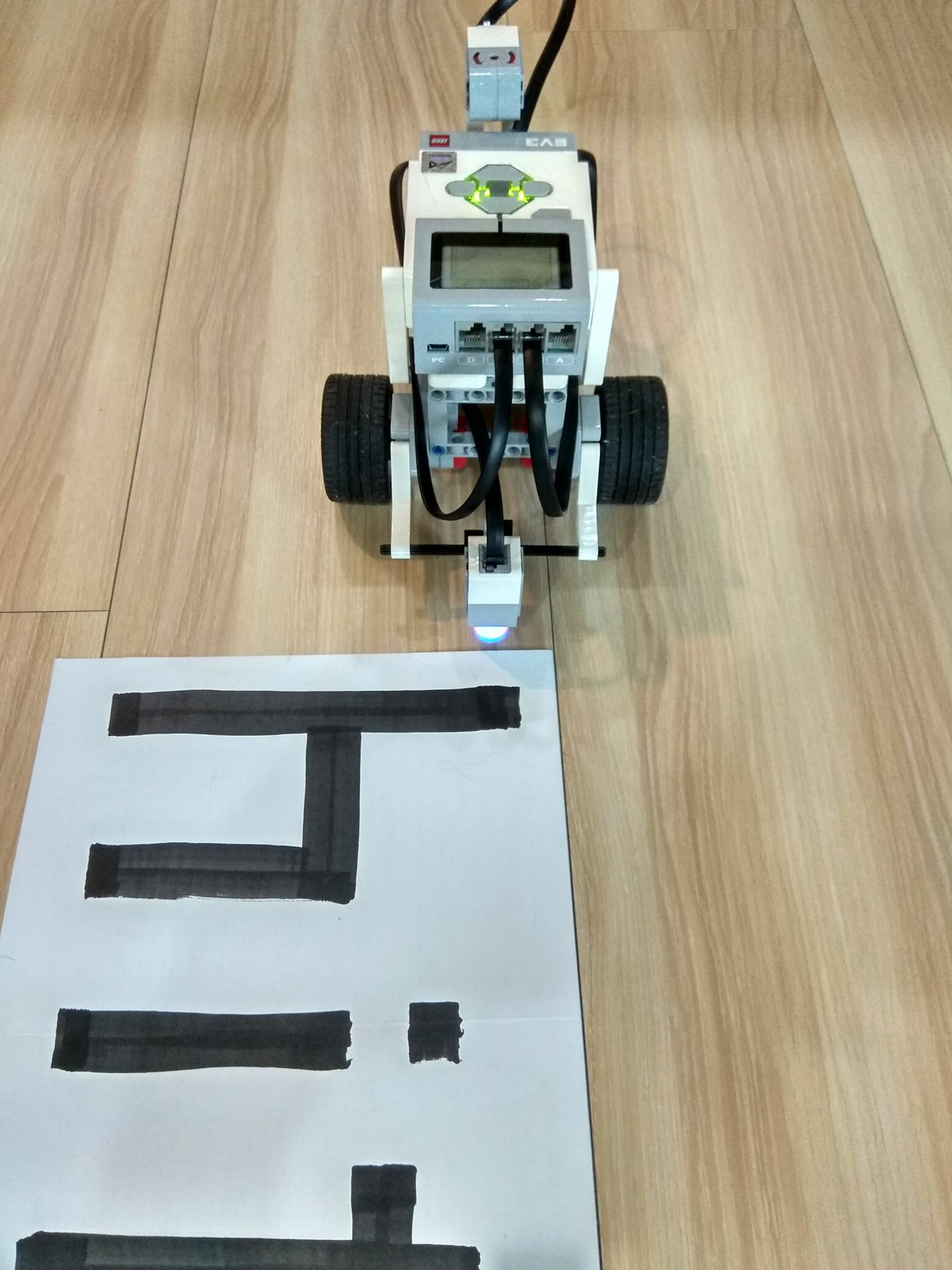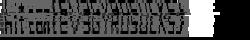# HITCON 2018 - EV3 Scanner

MISC

## General problem description

Similar to the previous challenge we got two images (see below) and a pcap.## Solution

Like before we use the found wireshark dissector to see what happens. However this time we find way more relevant packages than before.After some filtering we identified, that the base station sends only four different commands:

1. `OUTPUT_TIME_SPEED`: go in a direction with a constant speed for given time
2. `OUTPUT_STEP_SYNC`: turn given "ticks" long
3. `OUTPUT_STEP_SPEED`: go in a direction given "ticks" long
4. `INPUT_DEVICE`: read the sensor value

According to a documentation the tachometer is divided into 360 counts/ticks for each degree one, which makes sense for the `OUTPUT_STEP_SPEED`, however the `OUTPUT_STEP_SYNC` is called with -200 and +200 which we identified to be 90°.

Basically the Mastermind is sent to go 48 seconds long, and scan every now and then. After the 48 seconds turn right/left (depending on which side of the flag it is) go a little in that direction, turn again and start a new 48 second long journey with scanning.

As we mentioned before the export function of the dissector didn't work well, so we wrote the following python script to go through the RFCOMM exports to recover the flag:

``````#!/usr/bin/env python3

import json
import numpy
from PIL import Image

def mapFromTo(x,a,b,c,d):
y=(x-a)/(b-a)*(d-c)+c
return y

with open('rfcomm1.json') as f:

op_output_time_speed = 0xaf
op_output_step_sync = 0xb0
op_output_step_speed = 0xae
op_input_device = 0x99

image = [[], [], [], [], [], [], [], [], [], [], [], [], []]
current_row = -1
#reverse = True
reverse = False
min_val = 1000000000000000000000000
max_val = 0
turned = 0
ignore_next_time = False

# go through every RFCOMM package
for package in packages:
data = package['_source']['layers']['data']['data.data'].split(':')

# parse the relevant package fields
data_len = (int(data, 16) << 8) + int(data, 16)
msg_number = (int(data, 16) << 8) + int(data, 16)
cmd_type = int(data, 16)
if cmd_type == cmd_direct_command_reply :
variables = (int(data, 16) << 8) + int(data, 16)
op = int(data, 16)

# we ignore every response from commands which are not
# explicitly query the sensor, and a weak attempt to ignore
# scans which are not in the 48s timeframe
if op == op_input_device and turned == 0:
else:

if op == op_output_step_sync:
turned = (turned + 1) % 2

# we know that the output_time_speed command is issued for
# every new row...except for the 5th row, where after 6 scans the
# command was sent again for unkwown reasons
if op == op_output_time_speed and not ignore_next_time:
current_row += 1
if current_row == 4:
ignore_next_time = True
#reverse = not reverse
elif op == op_output_time_speed and ignore_next_time:
ignore_next_time = False

# parse and map the sensor data to float values
elif cmd_type == cmd_direct_reply and not ignore_next_reply:
val = (int(data, 16) << 24) + (int(data, 16) << 16) + (int(data, 16) << 8) + int(data, 16)
if val > max_val:
max_val = val
if val < min_val:
min_val = val
val = mapFromTo(val, 1065353216, 1610678784, 0, 1.0)

if reverse:
image[current_row].insert(0, val)
else:
image[current_row].append(val)

# pad every row to 250 columns to make pillow happy
for a in range(len(image)):
image[a] += [0.0] * (250 - len(image[a]))

# add every line 1500 times again to have bigger resolution
# as the 13*250 is not human friendly size
new_image = []
for a in image:
for b in range(1500):
new_image.append(a)

# show the array as image
np_image = numpy.asarray(new_image)
im = Image.fromarray(numpy.uint8(np_image * 255), 'L')
im.show()
``````

The script recovered a reasonable part of the image, but we needed some human intervention with gimp/paint to recover the flag.The flag was `hitcon{EV3GYROSUCS}`.